# Design two digital FIR LPF using Rectangular and Hamming windows, find filter's impulse response coefficients as wel...

Design two digital FIR LPF using Rectangular and Hamming windows, find filter's impulse response coefficients as well as their frequency responses. Filter specifications are: Cut-off frequency (ωp) = 1 radian, Filter Order (M) = 7.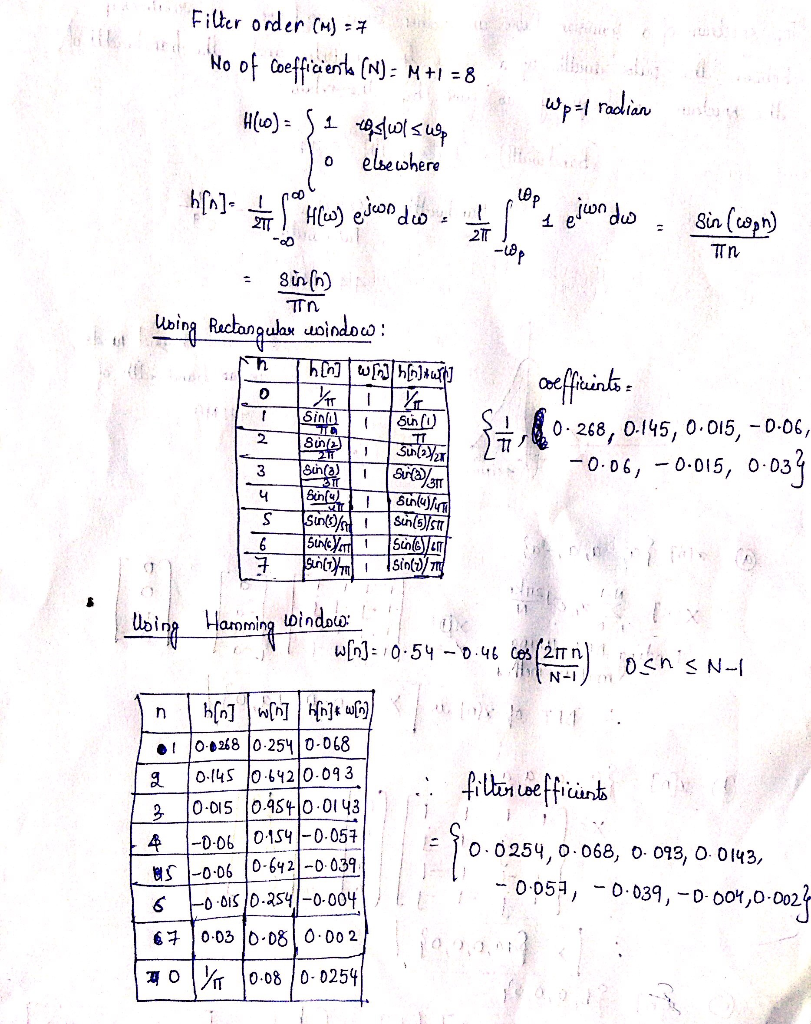##### Add Answer of: Design two digital FIR LPF using Rectangular and Hamming windows, find filter's impulse response coefficients as wel...
Similar Homework Help Questions
• ### b) When designing a FIR filters, the impulse response of the ideal low-pass filter is usually modified by multiplying i...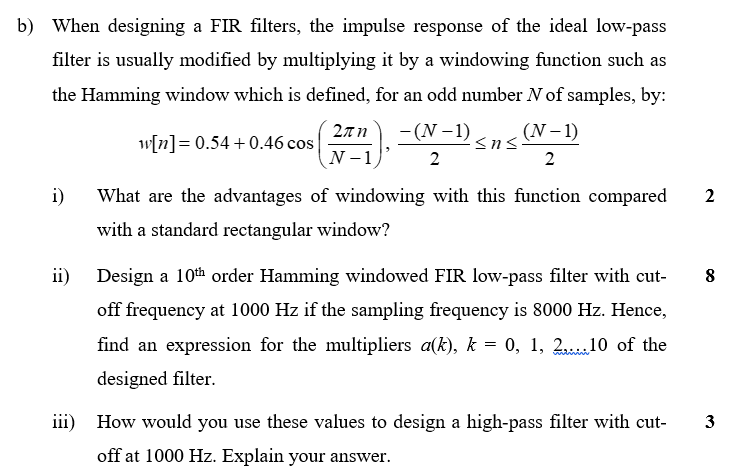b) When designing a FIR filters, the impulse response of the ideal low-pass filter is usually modified by multiplying it by a windowing function such as the Hamming window which is defined, for an odd number N of samples, by: (2n)-(N-I)-ns(N-1) N-12 wlnl 0.54 + 0.46 cos i What are the advantages of windowing with this function compared 2 with a standard rectangular window? ii) Design a 10th Order Hamming windowed FIR low-pass filter with cut- off frequency at 1000...

• ### 0.09 Rect Bartlett Hann 21 26 0.0063 44 amming0.0022 53 74 M+1 M1 +1 M+1 0.05 12π ckman0.0002 Figure 2: The characteristics of the window types. . FIR filter design Using the windowing method, design...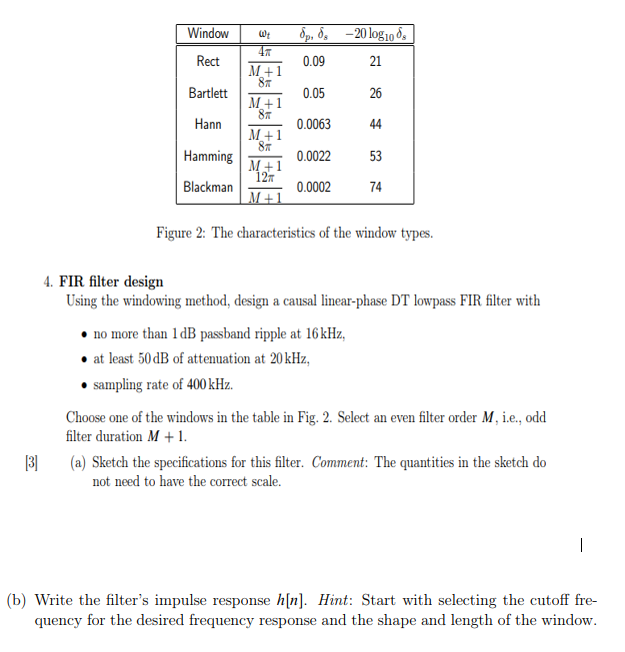0.09 Rect Bartlett Hann 21 26 0.0063 44 amming0.0022 53 74 M+1 M1 +1 M+1 0.05 12π ckman0.0002 Figure 2: The characteristics of the window types. . FIR filter design Using the windowing method, design a causal linear-phase DT lowpass FIR filter with no more than 1 dB passband ripple at 16kHz, at least 50dB of attenuation at 20kHz, sampling rate of 400 kHz. Choose one of the windows in the table in Fig. 2. Select an even filter order...

• ### Question 3 a) A linear-phase, Finite Impulse Response (FIR) digital filter with the transfer func...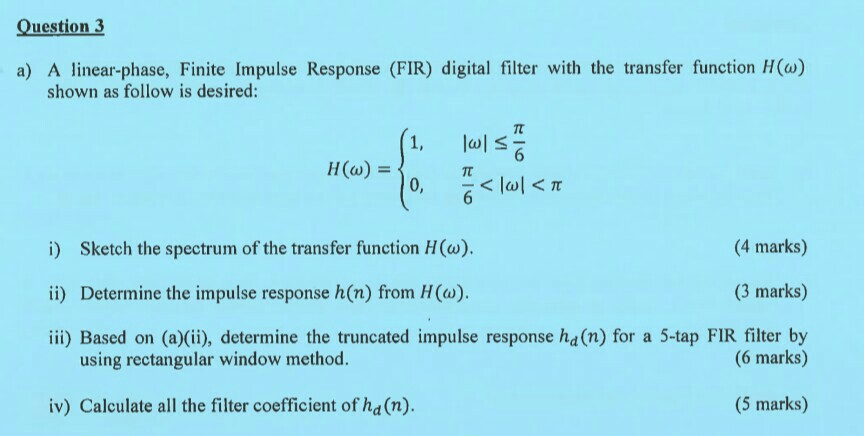Thanks Question 3 a) A linear-phase, Finite Impulse Response (FIR) digital filter with the transfer function H() shown as follow is desired: (4 marks) (3 marks) iii) Based on (a)(ii), determine the truncated impulse response ha(n) for a 5-tap FIR filter by i) Sketch the spectrum of the transfer function H (w). ii) Determine the impulse response h(n) from H() using rectangular window method. (6 marks) iv) Calculate all the filter coefficient of ha (n). (5 marks) Question 3 a)...

• ### QUESTION 6 Зро Design a second-order IIR digital low-pass filter using Butterworth approximation....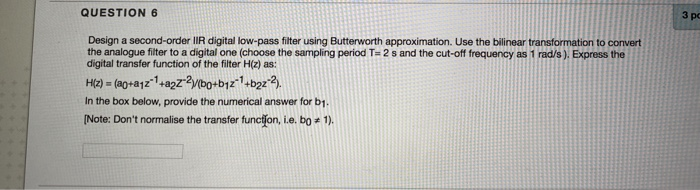QUESTION 6 Зро Design a second-order IIR digital low-pass filter using Butterworth approximation. Use the bilinear transformation to convert the analogue fiter to a digital one (choose the sampling period T- 2 s and the cut-off frequency as 1 rad/'s). Express the digital transfer function of the filter H(z) as: In the box below, provide the numerical answer for b1. [Note: Don't normalise the transfer func on, i.e. b0 # 1). r98111acontentid1837836_1&step QUESTION 7 Windowing based FIR filter design techniques...

• ### 1. Find the length of the lowpass FIR filter corresponding to the following specifications: wp- 0...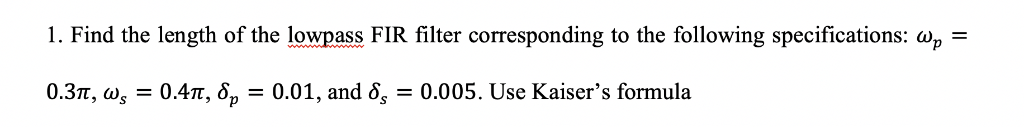1. Find the length of the lowpass FIR filter corresponding to the following specifications: wp- 0.3m ωs-0.4m, δp-0.01, and δ,-0.005. Use Kaiser's formula 4. Consider the design of a windowed FIR lowpass filter corresponding to the specifications given in problem #1. Determine its length if Hann, Hamming, and Blackman windows are used. Hint: refer to Equation 10.36 and Table 10.2 of the textbook. 5. With reference to the specifications in problem #1, consider the design of an FIR lowpass filter...

• ### QUESTION 28 3 points Save The Siter coefficients of a second-order digital IR filter are: ao-1,a1-2, a2-2, bo-1. b1-1/2, b2 1/8. (a's are numerator coetficients and b's are the denom...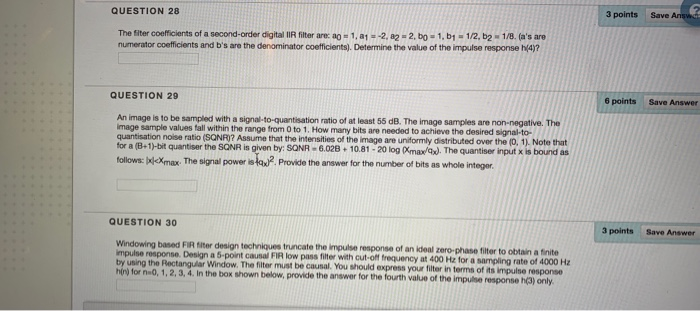QUESTION 28 3 points Save The Siter coefficients of a second-order digital IR filter are: ao-1,a1-2, a2-2, bo-1. b1-1/2, b2 1/8. (a's are numerator coetficients and b's are the denominator coefficients). Determine the value of the impulse response N4? QUESTION 29 6 points Save Answer An image is to be sampled with a signal-to-quantisation ratio of at least 55 dB. The image samples are non-negative. The image sample values fall within the range from 0 to 1. How many bits...

• ### 1. Design a custom FIR band-pass filter using the Fourier series and the Hanning window. The...

1. Design a custom FIR band-pass filter using the Fourier series and the Hanning window. The filter should be of order 8. We need to pass the signal in two audio bands 400-1600Hz and 4000-8000Hz and attenuate it elsewhere. The sampling frequency is 20 kHz. a) Calculate with pencil and paper the impulse response of the filter and the numerical values of the coefficients.

• ### 4. a) The sequence x[n] is related to its discrete time Fourier transform (DTFT). Xeo), by the expression: 27T i) Use t...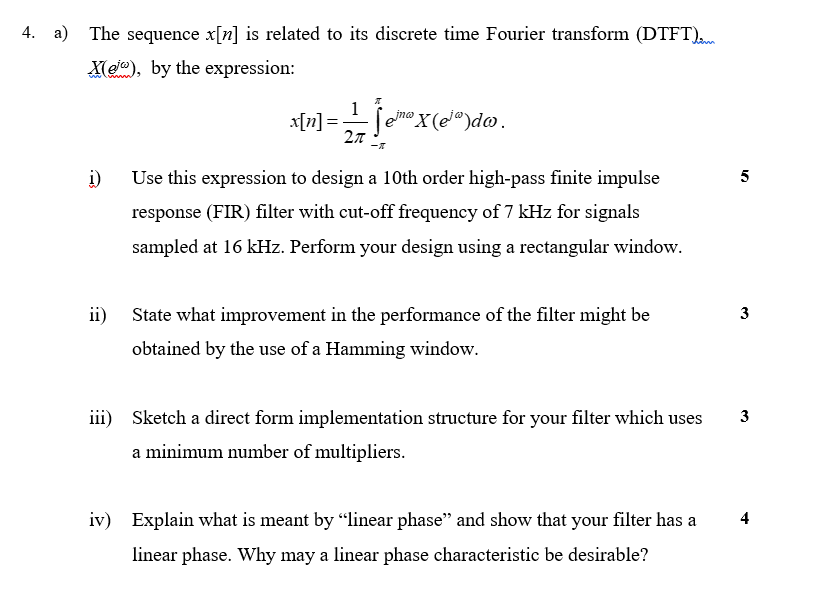4. a) The sequence x[n] is related to its discrete time Fourier transform (DTFT). Xeo), by the expression: 27T i) Use this expression to design a 10th order high-pass finite impulse response (FIR) filter with cut-off frequency of 7 kHz for signals sampled at 16 kHz. Perform your design using a rectangular window. ii State what improvement in the performance of the filter might be 3 obtained by the use of a Hamming window. iii) Sketch a direct form implementation...

• ### EE 448 Homework #6 1. Determine the impulse response, h(n), and plot the magnitude frequency response...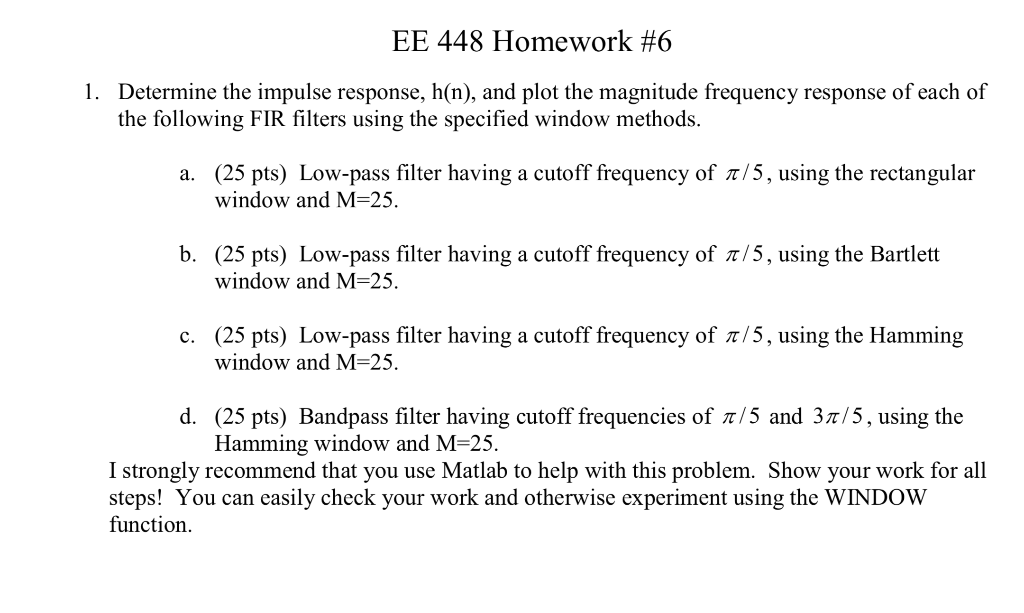EE 448 Homework #6 1. Determine the impulse response, h(n), and plot the magnitude frequency response of each of the following FIR filters using the specified window methods. (25 pts) Low-pass filter having a cutoff frequency of /5, using the rectangular window and M-25 a. b. (25 pts) Low-pass filter having a cutoff frequency of z/5, using the Bartlett window and M=25 (25 pts) Low-pass filter having a cutoff frequency of /5, using the Hamming window and M-25 c. d....

• ### Design lowpass IIR filter with the following specifications: Filter order = 2, Butterworth type C...

Design lowpass IIR filter with the following specifications: Filter order = 2, Butterworth type Cut-off frequency=800 Hz Sampling rate =8000 Hz Design using the bilinear z-transform design method Print the lowpass IIR filter coefficients and plot the frequency responses using MATLAB. MATLAB>>freqz(bLP,aLP,512,8000); axis([0 4000 –40 1]); Label and print your graph. What is the filter gain at the cut-off frequency 800 Hz? What are the filter gains for the stopband at 2000 Hz and the passband at 50 Hz based...

Need Online Homework Help?